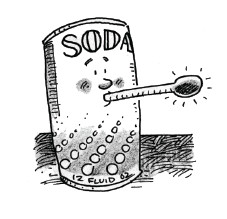### Home > CALC > Chapter 1 > Lesson 1.2.4 > Problem1-74

1-74.

A can of soda is $42°$F when purchased. Over the course of the next few hours, the temperature of the soda slowly rises. During an experiment, Shibisha used a thermometer and recorded the temperature at various times, $t$, shown in the table below. .

 time(min) temp (°F) $0$ $10$ $30$ $45$ $60$ $75$ $80$ $42$ $51$ $58$ $63$ $66$ $68$ $69$1. Sketch a graph of this temperature over time.

First plot the data. Then connect the points as smoothly as possible. How do we know that this will be a continuous graph?

2. When is the temperature changing the fastest? How does the graph tell you this?

Consider the relationship between rates of change and slope. Between which two points is the slope the greatest?

3. Approximately how fast is the temperature changing during the first $10$ minutes? How can you tell?

Use the eTool below to view the graph.
Click the link at right for the full version of the eTool: Calc 1-74 HW eTool